# subsequence sum

## Davenport's constant ★★★

Author(s):

For a finite (additive) abelian group, the Davenport constant of, denoted, is the smallest integerso that every sequence of elements ofwith length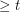has a nontrivial subsequence which sums to zero.

Conjecture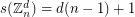Keywords: Davenport constant; subsequence sum; zero sum

## Gao's theorem for nonabelian groups ★★

Author(s): DeVos

For every finite multiplicative group, let() denote the smallest integerso that every sequence ofelements ofhas a subsequence of length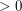(length) which has product equal to 1 in some order.

Conjecturefor every finite group.

Keywords: subsequence sum; zero sum

## Few subsequence sums in Z_n x Z_n ★★

Conjecture   For every, the sequence in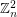consisting of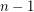copes of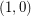andcopies ofhas the fewest number of distinct subsequence sums over all zero-free sequences fromof length.